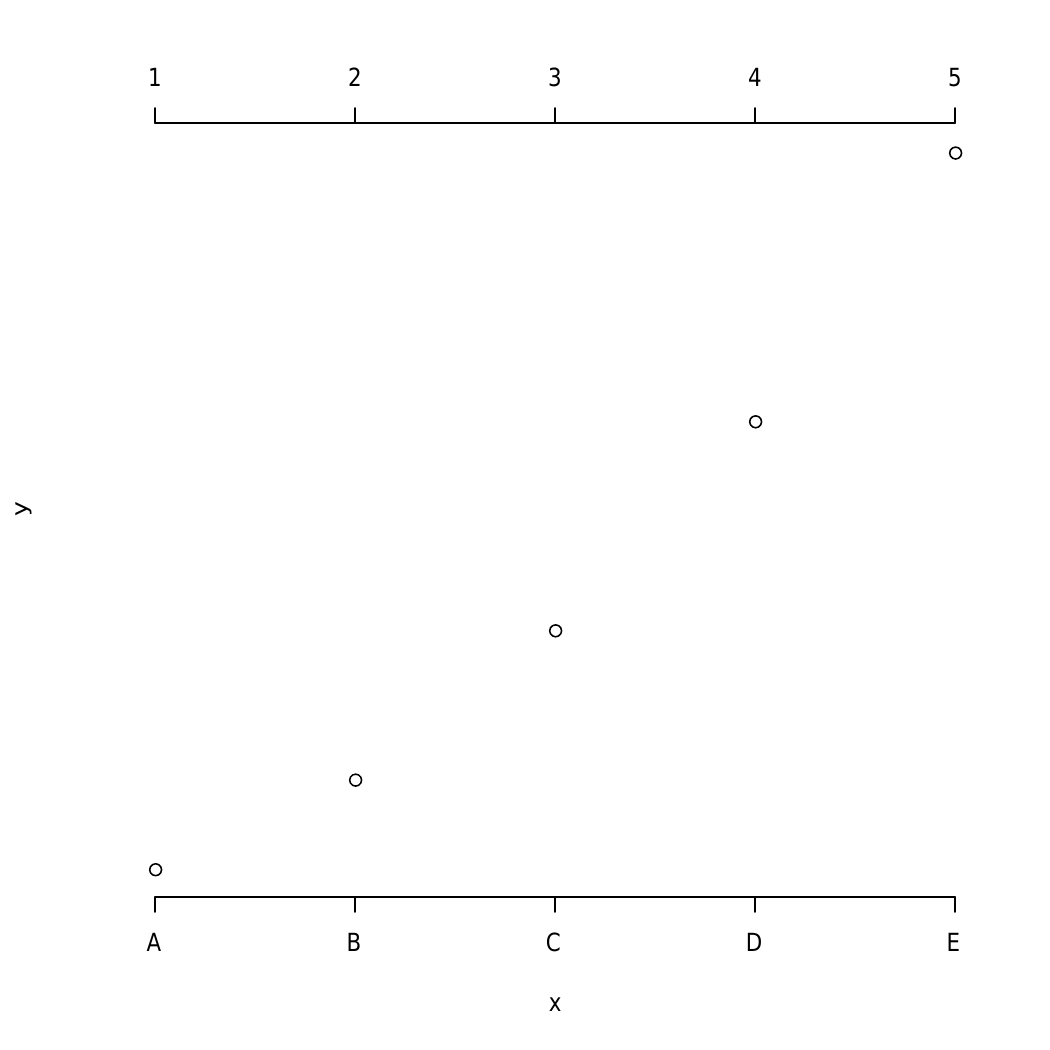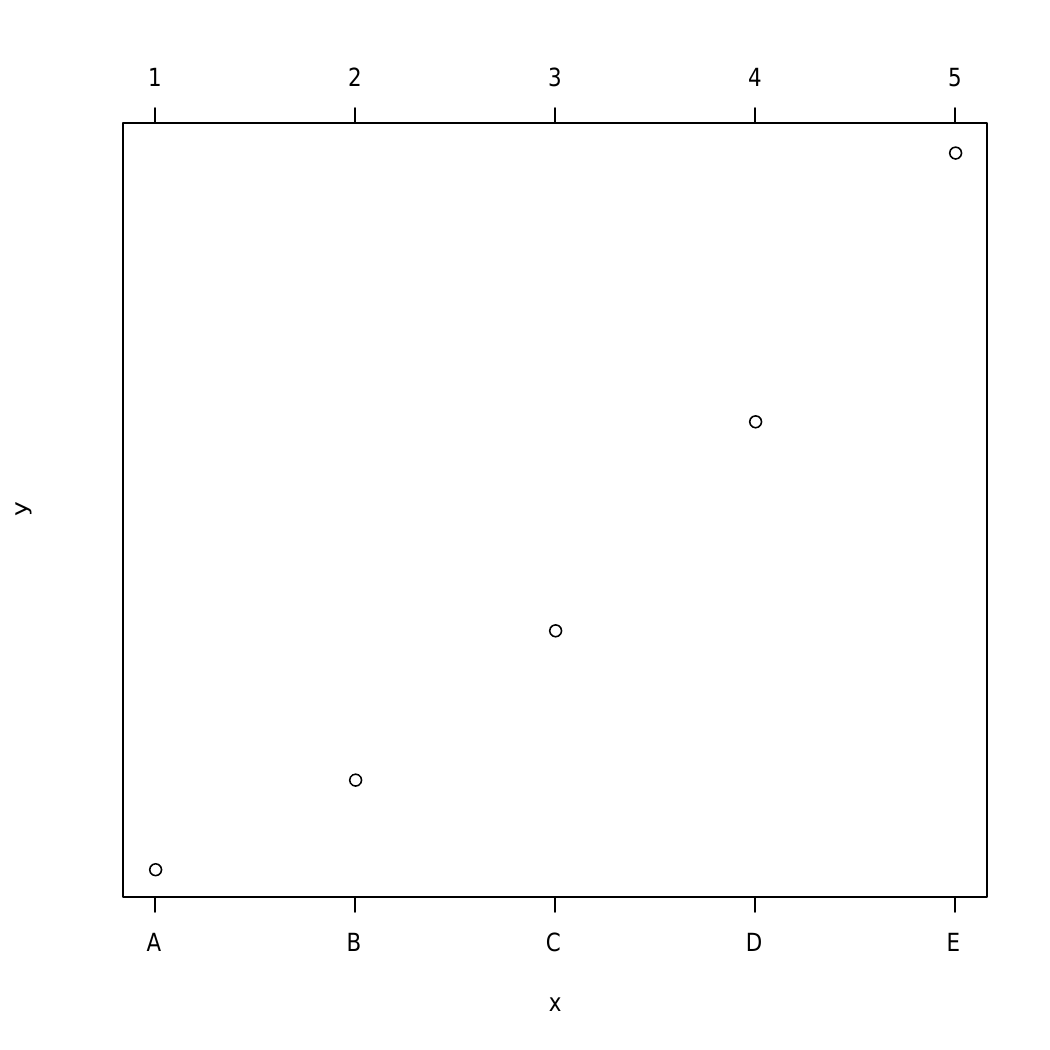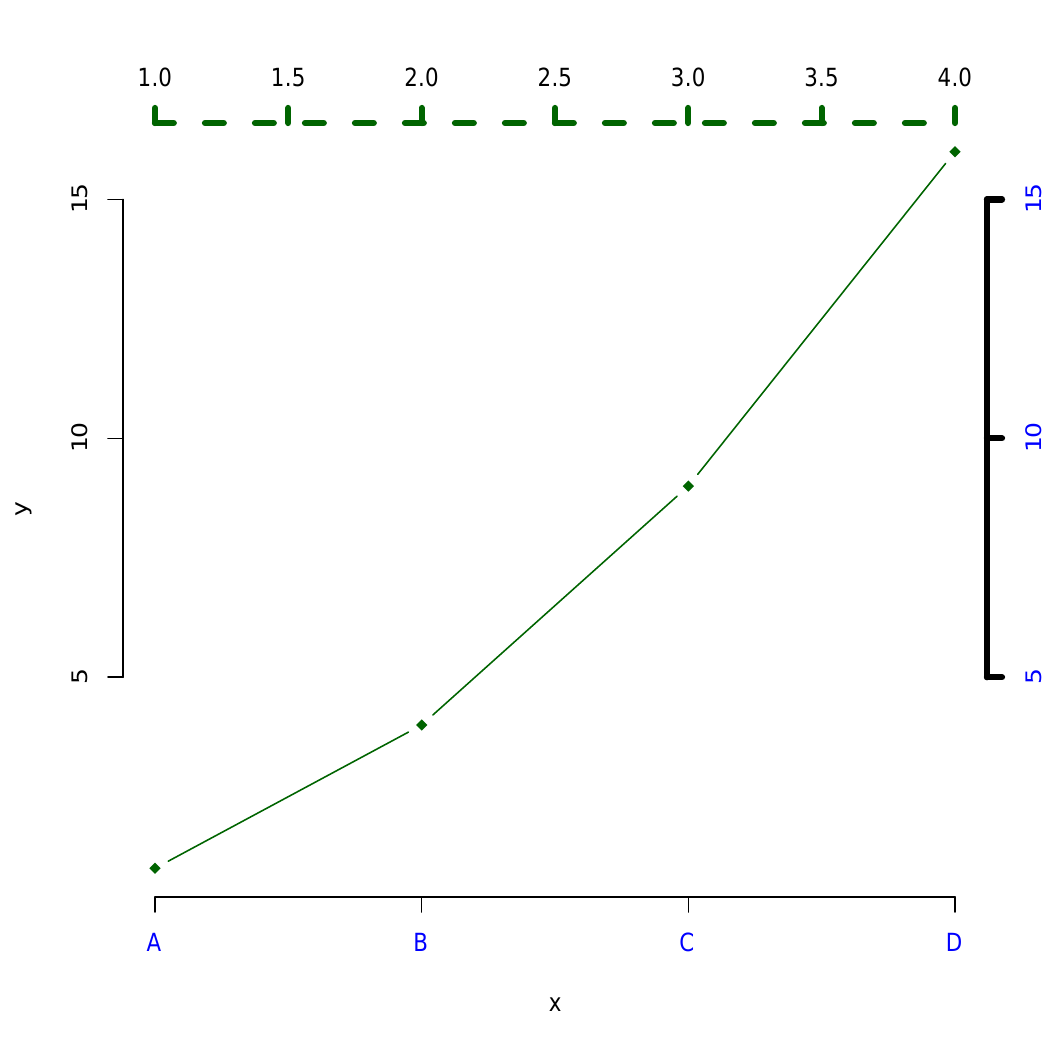Open In App

# Adding axis to a Plot in R programming – axis () Function

`axis()` function in R Language is to add axis to a plot. It takes side of the plot where axis is to be drawn as argument.

Syntax:
axis(side, at=NULL, labels=TRUE)

Parameters:
side: It defines the side of the plot the axis is to be drawn on possible values such as below, left, above, and right.
at: Point to draw tick marks
labels: Specifies texts for tick-mark labels.

Example 1:

 `# R program to draw axis in a plot``x <- 1:5; y = x * x``plot``(x, y, axes = ``FALSE``)`` ` `# Calling the axis() function``axis``(side = 1, at = 1:5, labels = ``LETTERS``[1:5])``axis``(3)`

Output:In the above example, the straight dotted line is added to the plot joining numerical and alphabetical values plot axis is drawn at the top and bottom part of the plot.

Example 2: Another example to add axes by drawing box

 `# R program to draw axis to a plot``x<-1:5; y = x * x``plot``(x, y, axes = ``FALSE``)``axis``(side=1, at = 1:5, labels = ``LETTERS``[1:5])``axis``(3)`` ` `#- To make it look like "usual" plot``box``() `

Output:Here, a box is drawn to drawn using box() function around the axis so that it looks like a usual plot.

Example 3:

 `# R program to draw axis to a plot``x<-1:4; y=x*x``plot``(x, y, pch = 18, col = ``"darkgreen"``, type = ``"b"``,``           ``frame = ``FALSE``, xaxt = ``"n"``) `` ` `# Remove x axis``axis``(1, 1:4, ``LETTERS``[1:4], col.axis=``"blue"``)``axis``(3, col = ``"darkgreen"``, lty = 2, lwd = 4)``axis``(4, col = ``"black"``, col.axis = ``"blue"``, lwd = 4)`

Output:Here, the different type of axis are drawn implementing modification in line width(lwd) and line type(lty).